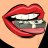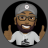# Vertical RSI-Based Time/Price-Change Zone#### Huffmac90

##### Member
Gents,

Playing with a new entry exit code that works pretty well but creates a terrible blob cloud across the screen. Trying to get it to just plot a vertical line where the cloud starts and stops.

Code:
``````def RSI = reference RSI(length = 13).RSI;
def highRSI = Highest(RSI, 21);
def lowRSI = Lowest(RSI, 21);
def RSIAverage = 100 * (RSI - lowRSI) / (highRSI - lowRSI);
def Net = Average(RSIAverage, 3);
def Delta = Average(Net, 5);
def Profile1 = highestall(high);
def Profile2 = lowestall(low);
def neg = if delta < delta then lowestall(low) else Double.NaN;

#plot P1plot = profile1;
#plot P2plot = Profile2;
#Plot negplot = neg;

D

#### diazlaz

##### Well-known member
2019 Donor
VIP
Hi @Huffmac90,

interesting. how would you use It ?

see this helps:

Ruby:
``````def RSI = reference RSI(length = 13).RSI;
def highRSI = Highest(RSI, 21);
def lowRSI = Lowest(RSI, 21);
def RSIAverage = 100 * (RSI - lowRSI) / (highRSI - lowRSI);
def Net = Average(RSIAverage, 3);
def Delta = Average(Net, 5);
def Profile1 = highestall(high);
def Profile2 = lowestall(low);
def neg = if delta < delta then lowestall(low) else Double.NaN;

def sState = if isNaN(neg) then 100 else -100;
AddVerticalLine(sState != sState and sState == 100, profile2, Color.green, curve.SHORT_DASH);
AddVerticalLine(sState != sState and sState == -100, profile1, Color.red, curve.SHORT_DASH);``````

J

#### john3

##### Active member
2019 Donor
@Huffmac90 Haven't seen this before. Interesting.

Did you come up with it on your own? If not, where have you seen it?#### Huffmac90

##### Member
@john3 something I saw someone in a blog post ask about doing so I figured what the hell and gave it a shot. it's pretty easy to use for people that want to trade in time chunks.Sync of price on vertical axis with Active Trader price Questions 5Create a Vertical line for current time with this code Questions 8